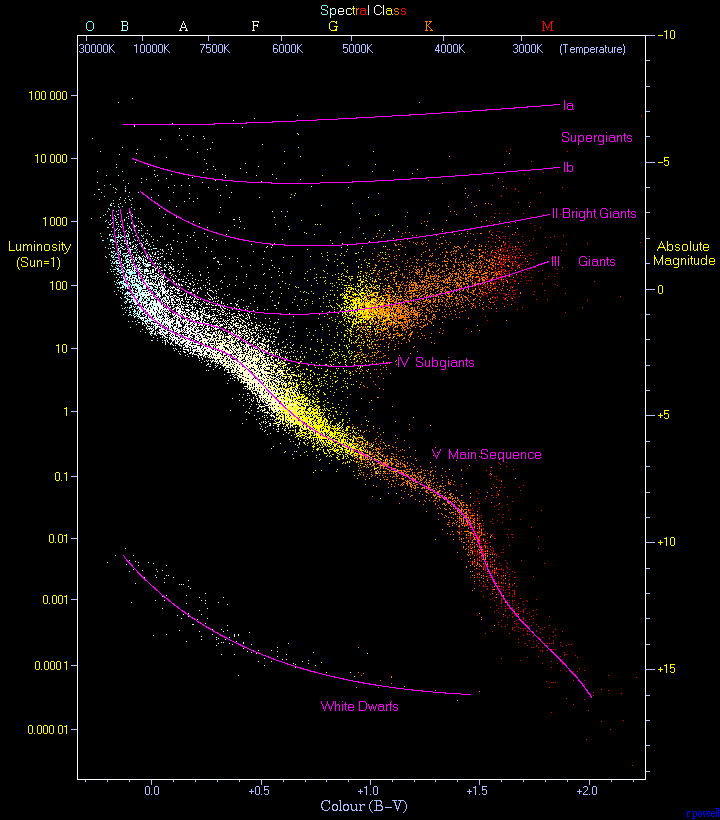# 11.1: Introduction

$$\newcommand{\vecs}{\overset { \rightharpoonup} {\mathbf{#1}} }$$ $$\newcommand{\vecd}{\overset{-\!-\!\rightharpoonup}{\vphantom{a}\smash {#1}}}$$$$\newcommand{\id}{\mathrm{id}}$$ $$\newcommand{\Span}{\mathrm{span}}$$ $$\newcommand{\kernel}{\mathrm{null}\,}$$ $$\newcommand{\range}{\mathrm{range}\,}$$ $$\newcommand{\RealPart}{\mathrm{Re}}$$ $$\newcommand{\ImaginaryPart}{\mathrm{Im}}$$ $$\newcommand{\Argument}{\mathrm{Arg}}$$ $$\newcommand{\norm}{\| #1 \|}$$ $$\newcommand{\inner}{\langle #1, #2 \rangle}$$ $$\newcommand{\Span}{\mathrm{span}}$$ $$\newcommand{\id}{\mathrm{id}}$$ $$\newcommand{\Span}{\mathrm{span}}$$ $$\newcommand{\kernel}{\mathrm{null}\,}$$ $$\newcommand{\range}{\mathrm{range}\,}$$ $$\newcommand{\RealPart}{\mathrm{Re}}$$ $$\newcommand{\ImaginaryPart}{\mathrm{Im}}$$ $$\newcommand{\Argument}{\mathrm{Arg}}$$ $$\newcommand{\norm}{\| #1 \|}$$ $$\newcommand{\inner}{\langle #1, #2 \rangle}$$ $$\newcommand{\Span}{\mathrm{span}}$$$$\newcommand{\AA}{\unicode[.8,0]{x212B}}$$

## Astronomy Laboratory 11 – The H-R Diagram

### Module IntroductionHertzsprung-Russell diagram, or H-R diagram. Some 22,000 stars make up the data for this specific illustration. HRDiagram by Richard Powell is licensed under CC BY-SA 2.5

The Hertzsprung-Russell Diagram, H-R Diagram, is a useful tool which plots stellar luminosity versus temperature. Building on the Star Colors and Spectroscopy lab, you will detail how the H-R Diagram works, and plot some of the stars observed in Lab 10, Star Colors and Spectroscopy. (1)

#### Objectives

At the end of this module, students will be able to:

• Plot a H-R diagram using provided data
• Analyze the data and make interpretations using the H-R diagram (1)

#### Outcomes

The material in this module includes content designed to meet the following course outcomes:

• Explain and apply major concepts in astronomy including planets, satellites, stars, meteors, galaxies, and theories of the universe.
• Demonstrate knowledge of scientific method.
• Communicate scientific ideas through oral or written assignments.
• Interpret scientific models such as formulas, graphs, tables and schematics, draw inferences from them and recognize their limitations.
• Demonstrate the ability to think critically.
• Demonstrate the ability to use scientific and quantitative reasoning. (1)